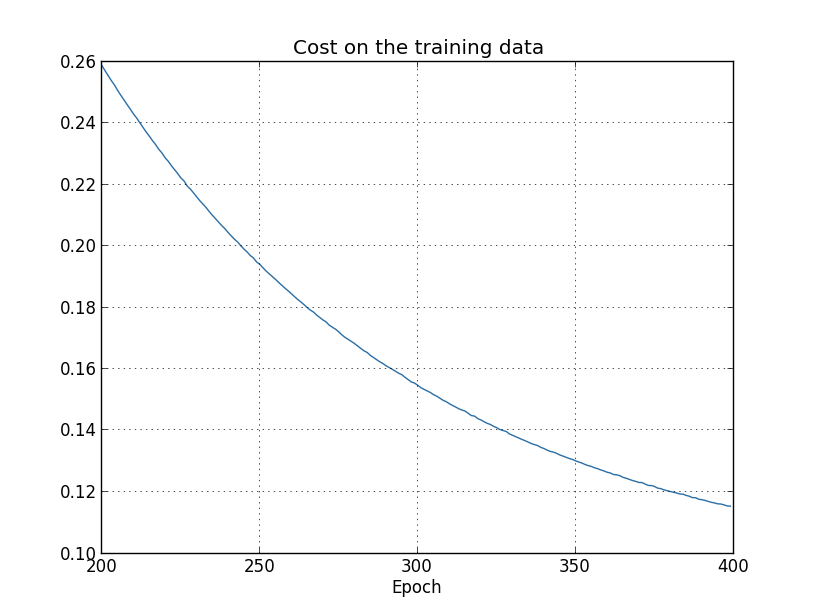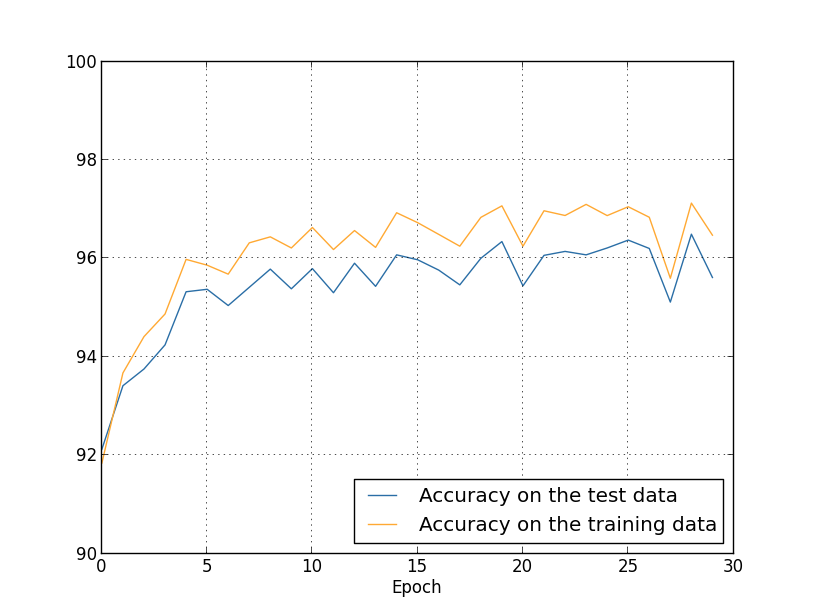# 正则化

>>> import mnist_loader
>>> training_data, validation_data, test_data = \
>>> import network2
>>> net = network2.Network([784, 30, 10](), cost=network2.CrossEntropyCost)
>>> net.large_weight_initializer()
>>> net.SGD(training_data[:1000](), 400, 10, 0.5,
... evaluation_data=test_data, lmbda = 0.1,
... monitor_evaluation_cost=True, monitor_evaluation_accuracy=True,
... monitor_training_cost=True, monitor_training_accuracy=True)>>> net.large_weight_initializer()
>>> net.SGD(training_data, 30, 10, 0.5,
... evaluation_data=test_data, lmbda = 5.0,
... monitor_evaluation_accuracy=True, monitor_training_accuracy=True)>>> net = network2.Network([784, 100, 10](),\
... cost=network2.CrossEntropyCost)
>>> net.large_weight_initializer()
>>> net.SGD(training_data, 30, 10, 0.5, lmbda=5.0,\
... evaluation_data=validation_data,\
... monitor_evaluation_accuracy=True)


1 这张图和下两张图的结果是由程序 overfitting.py 产生的。